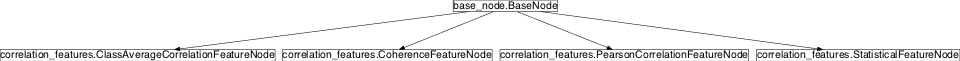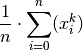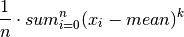# correlation_features¶

## Module: missions.nodes.feature_generation.correlation_features¶

Extract statistical properties like moments or correlation coefficients

Known issues
No unit tests!

Inheritance diagram for pySPACE.missions.nodes.feature_generation.correlation_features:## Class Summary¶

 StatisticalFeatureNode([raw_moment_order, ...]) Compute statistical features, e.g. PearsonCorrelationFeatureNode([segments, ...]) Compute pearson correlation of all pairs of channels ClassAverageCorrelationFeatureNode([segments]) Compute pearson correlation between channel segments and class averages CoherenceFeatureNode([frequency_band, ...]) Compute pairwise coherence of two channels with matplotlib.mlab.cohere

## Classes¶

### StatisticalFeatureNode¶

class pySPACE.missions.nodes.feature_generation.correlation_features.StatisticalFeatureNode(raw_moment_order=1, central_moment_order=1, std=False, std_function=None, quadratic_mean=False, median=False, minimum=False, maximum=False, artifact_threshold=None, **kwargs)[source]

Compute statistical features, e.g. central/raw moments, median, min etc.

This node computes statistical features like raw and central moments of k-th order:

1. raw_moment_of_k_th_order• k=1: mean;
• k=2: square root of value is quadratic mean
2. central_moment_of_k_th_order• k=1: 0;
• k=2: empirical variance;
• k=3: empirical skewness;
• k=4: kurtosis

In addition there could be computed: median, quadratic mean, standard deviation, minimum/maximum amplitude and the number of amplitudes above a given threshold.

Parameters

raw_moment_order:

The highest order (as an integer number) of raw moments that will be computed. For example:

0: no raw moment computation, mean mean and raw moment 2nd order etc.

Note

Values greater then 2 might take long computation time and are expected not to have any useful information.

(optional, default: 1)

central_moment_order:

The highest order (as an integer number) of central moments that will be computed. For example:

1: no central moment computation empirical variance empirical variance and empirical skewness

(optional, default: 1)

std:

True or False. If True the standard deviation of each channel is computed and retained as feature.

(optional, default: False)

std_function:

A string of a Python eval*able function. Specifies a function, e.g. numpy.std, if the algorithm should not use use the one in *standard_deviation. Specification of this parameter makes only sense if std is “True”.

(optional, default: None)

True or False. If True the square root of the second raw moment (quadratic mean) is computed and retained as feature.

(optional, default: False)

median:

True or False. If True the median of each channel is computed and retained as feature.

(optional, default: False)

minimum:

True or False. If True the minimum value of each channel is stored as a feature.

(optional, default: False)

maximum:

True or False. If True the maximum value of each channel is stored as a feature.

(optional, default: False)

artifact_threshold:

A float value. The number of amplitudes within a window above the given artifact_threshold is calculated and used as feature.

Note

The threshold depends on the actual resolution of the values. Check header file (for EEG data).

(optional, default: None)

Known issues

Exemplary Call

-
node : Statistical_Features
parameters :
central_moment_order : 0
std : True
median : True
artifact_threshold : 500.0

Author: Created: Anett Seeland (anett.seeland@dfki.de) 2009/10/06 2014/09/11 by Mario Michael Krell StatisticalFeatureNode Statistical_Features StatisticalFeature TimeSeries

Class Components Summary

 _execute(x) Calculates statistical features amplitudes_above(one_channel_time_series, ...) Return the number of values about the given threshold in the given time series. input_types raw_moment(one_channel_time_series, k) Compute the k’th-order raw moment of a given channel.
__init__(raw_moment_order=1, central_moment_order=1, std=False, std_function=None, quadratic_mean=False, median=False, minimum=False, maximum=False, artifact_threshold=None, **kwargs)[source]
raw_moment(one_channel_time_series, k)[source]

Compute the k’th-order raw moment of a given channel.

Arguments

one_channel_time_series:
a one dimensional time series
k:order of raw moment
amplitudes_above(one_channel_time_series, threshold)[source]

Return the number of values about the given threshold in the given time series.

Arguments

window: a tow dimensional time series a number above the values are count.
_execute(x)[source]

Calculates statistical features

input_types = ['TimeSeries']

### PearsonCorrelationFeatureNode¶

class pySPACE.missions.nodes.feature_generation.correlation_features.PearsonCorrelationFeatureNode(segments=1, max_segment_shift=0, *args, **kwargs)[source]

Compute pearson correlation of all pairs of channels

This node computes for all pairs of channels the Pearson product-moment correlation coefficient of certain time segments and returns each of correlation coefficient as feature.

Parameters

segments: max_segment_shift: The number of segments the time series window is split. (optional, default: 1) If 0, only the same segments of the two channels are compared. For n, each segment is also compared with the n,n-1,...,0 previous and later segments of the other channel. (optional, default: 0)

Exemplary Call

-
node : Pearson_Correlation_Features
parameters :
segments : 3
max_segment_shift : 2

Author: Created: Jan Hendrik Metzen (jhm@informatik.uni-bremen.de) 2009/03/18 Pearson_Correlation_Features PearsonCorrelationFeature PearsonCorrelationFeatureNode TimeSeries

Class Components Summary

__init__(segments=1, max_segment_shift=0, *args, **kwargs)[source]
_execute(x)[source]
input_types = ['TimeSeries']

### ClassAverageCorrelationFeatureNode¶

class pySPACE.missions.nodes.feature_generation.correlation_features.ClassAverageCorrelationFeatureNode(segments=1, *args, **kwargs)[source]

Compute pearson correlation between channel segments and class averages

This node computes for all channels the Pearson product-moment correlation coefficient between certain time segments and the class averages. Then each of the correlation coefficients is returned as feature.

Parameters

segments: The number of segments the time series window is split. (optional, default: 1)

Exemplary Call

-
node : Class_Average_Correlation_Features
parameters :
segments : 3

Author: Created: Jan Hendrik Metzen (jhm@informatik.uni-bremen.de) 2009/03/18 ClassAverageCorrelationFeatureNode Class_Average_Correlation_Features ClassAverageCorrelationFeature TimeSeries

Class Components Summary

 _execute(x) _stop_training() _train(x, label) input_types is_supervised() Returns whether this node is trainable is_trainable() Returns whether this node is trainable
__init__(segments=1, *args, **kwargs)[source]
is_trainable()[source]

Returns whether this node is trainable

is_supervised()[source]

Returns whether this node is trainable

_execute(x)[source]
_train(x, label)[source]
_stop_training()[source]
input_types = ['TimeSeries']

### CoherenceFeatureNode¶

class pySPACE.missions.nodes.feature_generation.correlation_features.CoherenceFeatureNode(frequency_band=None, frequency_resolution=None, *args, **kwargs)[source]

Compute pairwise coherence of two channels with matplotlib.mlab.cohere

Parameters

frequency_band: The frequency band which is used to extract features from the spectrogram. If None, the whole frequency band is used. (Optional, default: None)

Exemplary Call

-
node : Coherence_Features
parameters :
frequency_band : [0.4,3.5]

Author: Created: Jan Hendrik Metzen (jhm@informatik.uni-bremen.de) 2009/03/18 CoherenceFeatureNode CoherenceFeature Coherence_Features TimeSeries

Class Components Summary

__init__(frequency_band=None, frequency_resolution=None, *args, **kwargs)[source]
_execute(x)[source]
input_types = ['TimeSeries']